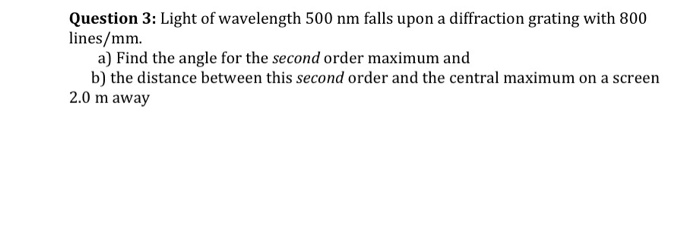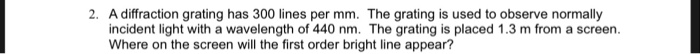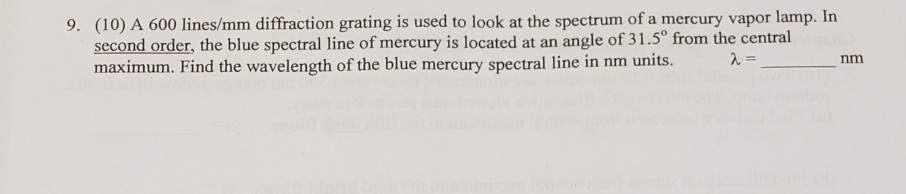Question

If the scientist uses a diffraction grating with 500 lines per mm and laser with a wavelength of 680 nm, what angle will a line from the grating to the second order bright spot make with the line from the grating to the central bright spot?

slit width * sin(theta) = order * wavelength

500 lines in 1 * 10^-3 m

1 line = 10^-3 / 500 m

slit width = 10^-3 / 500 m

10^-3 / 500 * sin(theta) = 2 * 680 * 10^-9

angle line from the grating to the second order bright spot theta = 42.84 degree

#### Earn Coins

Coins can be redeemed for fabulous gifts.

Similar Homework Help Questions
• ### If a diffraction grating produces a third-order bright spot for red light of wavelength 700 nm, at 65° from the central maximum at what angle will the second order bright spot be for violet light...If a diffraction grating produces a third-order bright spot for red light of wavelength 700 nm, at 65° from the central maximum at what angle will the second order bright spot be for violet light of wavelength 400 nm? How many lines per mm on this grating? If a diffraction grating produces a third-order bright spot for red light of wavelength 700 nm, at 65° from the central maximum at what angle will the second order bright spot be for...

• ### A 500 lines per mm diffraction grating is illuminated by light of wavelength 510...continues

A 500 lines per mm diffraction grating is illuminated by light of wavelength 510 nm. What is the angle of each diffraction order?

• ### A 500 lines per mm diffraction grating is illuminated by light of wavelength 560 nm ....

A 500 lines per mm diffraction grating is illuminated by light of wavelength 560 nm . Part A What is the maximum diffraction order seen Part B: What is the angle of each diffraction order starting from zero diffraction order to the maximum visible diffraction order? Enter your answers in degrees in ascending order separated by commas.

• ### Question 3: Light of wavelength 500 nm falls upon a diffraction grating with 800 lines/mm. a)...Question 3: Light of wavelength 500 nm falls upon a diffraction grating with 800 lines/mm. a) Find the angle for the second order maximum and b) the distance between this second order and the central maximum on a screen 2.0 m away

• ### 2. A diffraction grating has 300 lines per mm. The grating is used to observe normally...2. A diffraction grating has 300 lines per mm. The grating is used to observe normally incident light with a wavelength of 440 nm. The grating is placed 1.3 m from a screen. Where on the screen will the first order bright line appear?

• ### A 500 lines per mm diffraction grating is illuminated by light of wavelength 640 nm .

A 500 lines per mm diffraction grating is illuminated by light of wavelength 640 nm . For the steps and strategies involved in solving a similar problem, you may view a Video Tutor Solution.Part AWhat is the maximum diffraction order seen? Express your answer as an integer. Part B What is the angle of each diffraction order starting from zero diffraction order to the maximum visible diffraction order?

• ### Light from an argon laser strikes a diffraction grating that has 5,816 lines per cm. The...

Light from an argon laser strikes a diffraction grating that has 5,816 lines per cm. The central and first-order principal maxima are separated by 0.5 m on a wall 1.52 m from the grating. What is the wavelength of the laser light in nm? Enter an integer.

• ### A laser light was shone through a diffraction grating whose lines were 1/1000 mm apart. The...

A laser light was shone through a diffraction grating whose lines were 1/1000 mm apart. The distance was measured between the center spot and the first side spot andfound to be 99 mm. The distance from the diffraction grating to the first side spot was found to be 154 mm. Calculate the wavelength of light in nm that the laserpointer was emitting.

• ### A diffraction grating with 230 lines per mm is used in an experiment to study the...

A diffraction grating with 230 lines per mm is used in an experiment to study the visible spectrum of a gas discharge tube. At what angle from the beam axis will the first order peak occur if the tube emits light with wavelength of 421.2 nm? At what angle will the second order peak occur?

• ### 9. (10) A 600 lines/mm diffraction grating is used to look at the spectrum of a...9. (10) A 600 lines/mm diffraction grating is used to look at the spectrum of a mercury vapor lamp. In second order, the blue spectral line of mercury is located at an angle of 31.5° from the central maximum. Find the wavelength of the blue mercury spectral line in nm units.